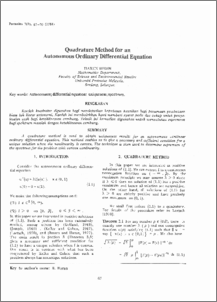# Quadrature Method for an Autonomous Ordinary Differential Equation

## Citation

Budin, Harun (1984) Quadrature Method for an Autonomous Ordinary Differential Equation. Pertanika, 7 (2). pp. 67-70.

## Abstract

A quadrature method is used to obtain uniqueness results for an autonomous nonlinear ordinary differential equation. This method enables us to give a necessary and sufficient condition for a unique solution when the nonlinearity is convex. The technique is then used to determine supremum of the spectrum for the problem with convex nonlinearity.Preview
PDFView Item